## What Is Ionic Migration?

Fluid Flow, Heat Transfer, and Mass Transport   Ionic Migration

### Understanding the Migration of Ions

Mathematical modeling and numerical simulations are used extensively to understand electrochemical systems and the migration of ions. The model equations that describe the migration of ions in electrolytes may be derived from relatively simple principles.Potential distribution in the electrolyte at the surface of a boat's hull, propellers, and the sacrificial anodes that protect the hull from corrosion. This simulation uses a model for the migration of ions in seawater. Modeling can be used to ensure that protection is obtained for different positions of the anodes.

Potential distribution in the electrolyte at the surface of a boat's hull, propellers, and the sacrificial anodes that protect the hull from corrosion. This simulation uses a model for the migration of ions in seawater. Modeling can be used to ensure that protection is obtained for different positions of the anodes.

In the absence of an external force, ions in an electrolyte are under endless random motion. The transport of ions under such conditions may be described by diffusion.

(1)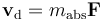In this constitutive relation, vd denotes the drift velocity (m s-1); mabs, the absolute mobility (N s m-1); and F, the applied force (N). The total migration flux of an ion, due to its drift velocity, is given by the product of its concentration and drift velocity. The total flux (mol m-2 s-1) of an ion, Ni, in the presence of both a concentration gradient and an applied force is the sum of the contributions from diffusion and migration:

(2)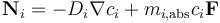where Di is the diffusion coefficient or diffusivity, and ci denotes concentration (mol m-3). If the force is adjusted to achieve a zero net flux, with equal and opposite diffusion and migration, we must have:

(3)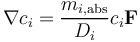However, under the condition of zero net flux in all directions, equilibrium may be assumed and the concentration distribution can be described by the Boltzmann equation :

(4)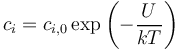where ci,0 denotes the concentration at zero potential energy and U represents the potential energy (J) of a molecule. The gradient of this concentration field then yields:

(5)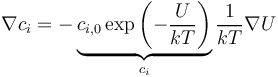where k is the Boltzmann constant.

Per definition, the force is the negative gradient of the potential energy:

(6)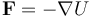The combination of equations 3 and 5 for the concentration gradient gives:

(7)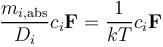which, by elimination, results in the Einstein relation for mobility :

(8)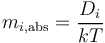This expression is extremely important, since it directly relates the mobility of an ion, subject to any force, to its diffusivity. This mobility can be used to estimate the flux of ions through migration and also the electrical conductivity of an electrolyte.Flux distribution in the direction along the main component of the electric field in an electrodialysis unit cell. At the position of the anion selective membrane, the migrative flux of sodium ions is perfectly balanced by its diffusive flux, so that the total flux over the membrane boundaries – on both interfaces of the membrane – is zero. The main flux of sodium ions is, therefore, to the left – over the cation-selective membrane. The opposite takes place for chloride, which fluxes to the right – across the anion-selective membrane. The electric field goes from right to left.

Flux distribution in the direction along the main component of the electric field in an electrodialysis unit cell. At the position of the anion selective membrane, the migrative flux of sodium ions is perfectly balanced by its diffusive flux, so that the total flux over the membrane boundaries – on both interfaces of the membrane – is zero. The main flux of sodium ions is, therefore, to the left – over the cation-selective membrane. The opposite takes place for chloride, which fluxes to the right – across the anion-selective membrane. The electric field goes from right to left.

According to the following equation, when an electric field is applied to the ions in an electrolyte, the directed force, introduced above, is proportional to the electric field:

(9)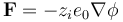where zi denotes the charge number of the ion, e0 is the elementary charge on an electron, φ is the electric potential (V), and −∇φ represents the electric field. The drift velocity is, therefore, given by:

(10)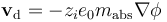We will define the electrochemical mobility of an ion i (m2 s-1 V-1), denoted ui, as the ratio of the magnitude of its drift velocity to that of the applied electric field:

(11)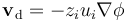Using the Einstein relation for mabs, we can now write out the Nernst-Einstein relation in which the electrochemical mobility is related to the diffusivity:

(11a)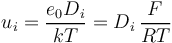Here, F is the Faraday constant and R is the gas constant. The resulting migration flux of an ion, i, is the product of the drift velocity with the ion’s concentration, ci. The contribution of this flux is referred to as migration of ions or electromigration:

(12)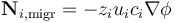The current density, i, in an electrolyte may be obtained from the sum of the contributions from all ions in the electrolyte:

(13)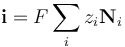Deposition thickness of paint on the surface of a car door during electrophoretic painting. The horizontal slice plot shows the potential assuming electroneutrality and constant composition in the cell. These types of simulations may be used to design and control the process.

Deposition thickness of paint on the surface of a car door during electrophoretic painting. The horizontal slice plot shows the potential assuming electroneutrality and constant composition in the cell. These types of simulations may be used to design and control the process.

In a general dilute electrolyte, the flux may have three contributions from diffusion, migration, and convection:

(14)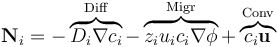where Di denotes the diffusivity and u is the velocity of the electrolyte. Substituting this flux expression into equation 13 for the current density gives the following:

(15)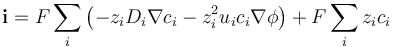In most electrochemical cells, with the exception of the charged double layer, electroneutrality can be assumed in the electrolyte:

(16)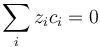This eliminates the last term in equation 15, which is the possible contribution of the convective term to the transport of current in an electrolyte. However, convection contributes by maintaining a uniform concentration distribution, and hence constant conductivity, everywhere in the cell except close to the electrodes, where electroactive species may be consumed at a high rate. For an electroneutral electrolyte at constant composition (zero concentration gradient), the expression for current density becomes:

(17)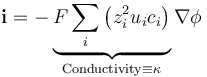where Κ is defined as the conductivity of the electrolyte. This equation shows that the electric current in electrolytes with constant composition is due solely to migration. The current obeys Ohm's law with a conductivity given by the sum of the contributions of each component ion in the electrolyte.

This relation is used in electrochemical engineering for describing primary and secondary current density distribution applications. It is also widely used for modeling electrolyte currents in general electrochemistry and electroanalytic chemistry.

Published: January 14, 2015